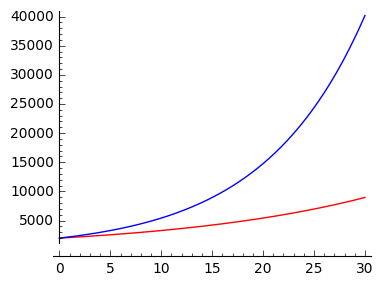# Money in a bank account grows continuously at an annual rate of r (when the interest rate is 5%,...

Money in a bank account grows continuously at an annual rate of r (when the interest rate is 5%, r = 0.05, and so on). Suppose 2000 is put into the account in 2010. (a) Write a differential equation satisfied by M, the amount of money in the account at time t, measured in years since 2010. (b) Solve the differential equation. (c) Sketch the solution until the year 2040 for interest rates of 5% and 10%. ## First-Order Linear Differential Equations: An equation of the form {eq}\dfrac{dy}{dt}+a(t)y=b(t) {/eq} is called a first-order linear differential equation for the unknown function {eq}y {/eq}. A function {eq}K(t)=e^{\int a(t) \, dt} {/eq} is called an integrating factor for this equation. This is because multiplying the equation by {eq}K(t) {/eq} will put it into a form where it can easily be integrated. ## Answer and Explanation: (a) If the account earns income at an annual rate of {eq}r {/eq}, then at any instant the rate of change in the amount of money in the account is {eq}rM {/eq} dollars per year. That is, if time is measured in years, the amount of money in the account satisfies the differential equation {eq}\boxed{\frac{dM}{dt}=rM}\, {/eq}. (b) The differential equation {eq}\frac{dM}{dt}=rM {/eq} is a first-order linear equation, so we can find its general solution as follows: {eq}\begin{align*} \frac{dM}{dt}&=rM\\ \frac{dM}{dt}-rM&=0&\text{(rewriting in standard form)}\\ e^{-rt}\frac{dM}{dt}-re^{-rt}M&=0&\text{(multiplying by the integrating factor }e^{\int -r \, dt}=e^{-rt}\text{)}\\ \frac{d}{dt}(e^{-rt}M)&=0&\text{(applying the product rule in reverse)}\\ e^{-rt}M&=C&\text{(integrating both sides of the equation; }C\text{ is a constant of integration)}\\ M&=Ce^{rt}&\text{(isolating }M\text{).} \end{align*} {/eq} We're given that the initial amount of money in the account is2000, so {eq}M(0)=2000 {/eq}. We can use this initial condition to solve for the constant {eq}C {/eq}:

{eq}\begin{align*} 2000&=M(0)\\ &=Ce^{r\cdot 0}\\ &=C \, . \end{align*} {/eq}

So the solution to the differential equation is {eq}\boxed{M=2000e^{rt}}\, {/eq}.

(c) In terms of our variable {eq}t {/eq}, the range of time from 2010 to 2040 corresponds to {eq}0 \le t \le 30 {/eq}. The graph of the above equation for 5% and 10% interest over this range is given below, with 5% interest in red and 10% interest in blue: